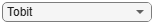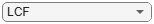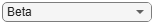# predict

Predict exposure at default

Since R2021b

## Syntax

``predictedEAD = predict(eadModel,data)``
``predictedEAD = predict(___,Name=Value)``

## Description

example

````predictedEAD = predict(eadModel,data)` computes the exposure at default (EAD). When using a `Regression` model, the `predict` function operates on the underlying compact statistical model and then transforms the predicted values back to the EAD scale.```

example

````predictedEAD = predict(___,Name=Value)` specifies options using one or more name-value arguments in addition to the input arguments in the previous syntax.```

## Examples

collapse all

This example shows how to use `fitEADModel` to create a `Tobit` model and then predict exposure at default (EAD) values.

```load EADData.mat head(EADData)```
``` UtilizationRate Age Marriage Limit Drawn EAD _______________ ___ ___________ __________ __________ __________ 0.24359 25 not married 44776 10907 44740 0.96946 44 not married 2.1405e+05 2.0751e+05 40678 0 40 married 1.6581e+05 0 1.6567e+05 0.53242 38 not married 1.7375e+05 92506 1593.5 0.2583 30 not married 26258 6782.5 54.175 0.17039 54 married 1.7357e+05 29575 576.69 0.18586 27 not married 19590 3641 998.49 0.85372 42 not married 2.0712e+05 1.7682e+05 1.6454e+05 ```
```rng('default'); NumObs = height(EADData); c = cvpartition(NumObs,'HoldOut',0.4); TrainingInd = training(c); TestInd = test(c);```

Select Model Type

Select a model type for `Tobit` or `Regression`.

`ModelType ="Tobit";`

Select Conversion Measure

Select a conversion measure for the EAD response values.

`ConversionMeasure ="LCF";`

Create `Tobit` EAD Model

Use `fitEADModel` to create a `Tobit` model using the `TrainingInd` data.

```eadModel = fitEADModel(EADData(TrainingInd,:),ModelType,PredictorVars={'UtilizationRate','Age','Marriage'}, ... ConversionMeasure=ConversionMeasure,DrawnVar="Drawn",LimitVar="Limit",ResponseVar="EAD"); disp(eadModel);```
``` Tobit with properties: CensoringSide: "both" LeftLimit: 0 RightLimit: 1 ModelID: "Tobit" Description: "" UnderlyingModel: [1x1 risk.internal.credit.TobitModel] PredictorVars: ["UtilizationRate" "Age" "Marriage"] ResponseVar: "EAD" LimitVar: "Limit" DrawnVar: "Drawn" ConversionMeasure: "lcf" ```

Display the underlying model. The underlying model's response variable is the transformation of the EAD response data. Use the `'LimitVar'` and `'DrawnVar'` name-value arguments to modify the transformation.

`disp(eadModel.UnderlyingModel);`
```Tobit regression model: EAD_lcf = max(0,min(Y*,1)) Y* ~ 1 + UtilizationRate + Age + Marriage Estimated coefficients: Estimate SE tStat pValue __________ __________ ________ __________ (Intercept) 0.22467 0.031679 7.092 1.6924e-12 UtilizationRate 0.4714 0.020683 22.791 0 Age -0.0014209 0.00077072 -1.8436 0.065356 Marriage_not married -0.010542 0.015811 -0.66676 0.50498 (Sigma) 0.3618 0.0049791 72.665 0 Number of observations: 2627 Number of left-censored observations: 0 Number of uncensored observations: 2626 Number of right-censored observations: 1 Log-likelihood: -1057.9 ```

EAD prediction operates on the underlying compact statistical model and then transforms the predicted values back to the EAD scale. You can specify the `predict` function with different options for the `'ModelLevel'` name-value argument.

```predictedEAD = predict(eadModel, EADData(TestInd,:),ModelLevel="ead"); predictedConversion = predict(eadModel, EADData(TestInd,:),ModelLevel="ConversionMeasure");```

This example shows how to use `fitEADModel` to create a `Beta` model and then predict exposure at default (EAD) values.

```load EADData.mat head(EADData)```
``` UtilizationRate Age Marriage Limit Drawn EAD _______________ ___ ___________ __________ __________ __________ 0.24359 25 not married 44776 10907 44740 0.96946 44 not married 2.1405e+05 2.0751e+05 40678 0 40 married 1.6581e+05 0 1.6567e+05 0.53242 38 not married 1.7375e+05 92506 1593.5 0.2583 30 not married 26258 6782.5 54.175 0.17039 54 married 1.7357e+05 29575 576.69 0.18586 27 not married 19590 3641 998.49 0.85372 42 not married 2.0712e+05 1.7682e+05 1.6454e+05 ```
```rng('default'); NumObs = height(EADData); c = cvpartition(NumObs,'HoldOut',0.4); TrainingInd = training(c); TestInd = test(c);```

Select Model Type

Select a model type for `Beta`.

`ModelType ="Beta";`

Select Conversion Measure

Select a conversion measure for the EAD response values.

`ConversionMeasure ="LCF";`

Create `Beta` EAD Model

Use `fitEADModel` to create a `Beta` model using `EADData`.

```eadModel = fitEADModel(EADData,ModelType,PredictorVars={'UtilizationRate','Age','Marriage'}, ... ConversionMeasure=ConversionMeasure,DrawnVar="Drawn",LimitVar="Limit",ResponseVar="EAD"); disp(eadModel);```
``` Beta with properties: BoundaryTolerance: 1.0000e-07 ModelID: "Beta" Description: "" UnderlyingModel: [1x1 risk.internal.credit.BetaModel] PredictorVars: ["UtilizationRate" "Age" "Marriage"] ResponseVar: "EAD" LimitVar: "Limit" DrawnVar: "Drawn" ConversionMeasure: "lcf" ```

Display the underlying model. The underlying model's response variable is the transformation of the EAD response data. Use the `'LimitVar'` and `'DrawnVar'` name-value arguments to modify the transformation.

`disp(eadModel.UnderlyingModel);`
```Beta regression model: logit(EAD_lcf) ~ 1_mu + UtilizationRate_mu + Age_mu + Marriage_mu log(EAD_lcf) ~ 1_phi + UtilizationRate_phi + Age_phi + Marriage_phi Estimated coefficients: Estimate SE tStat pValue __________ _________ _________ __________ (Intercept)_mu -0.6741 0.087775 -7.6799 1.954e-14 UtilizationRate_mu 1.6974 0.060621 28 0 Age_mu -0.0046006 0.0021317 -2.1582 0.030965 Marriage_not married_mu -0.0020533 0.040397 -0.050827 0.95947 (Intercept)_phi -0.43364 0.071602 -6.0562 1.5101e-09 UtilizationRate_phi 0.42461 0.051852 8.1889 4.4409e-16 Age_phi -0.0036089 0.0017592 -2.0514 0.040285 Marriage_not married_phi -0.016663 0.032976 -0.50531 0.61337 Number of observations: 4378 Log-likelihood: -5255.12 ```

EAD prediction operates on the underlying compact statistical model and then transforms the predicted values back to the EAD scale. You can specify the `predict` function with different options for the `'ModelLevel'` name-value argument.

```predictedEAD = predict(eadModel, EADData(TestInd,:),ModelLevel="ead"); predictedConversion = predict(eadModel, EADData(TestInd,:),ModelLevel="ConversionMeasure");```

## Input Arguments

collapse all

Exposure at default model, specified as a previously created `Regression`, `Tobit`, or `Beta` object using `fitEADModel`.

Data Types: `object`

Data, specified as a `NumRows`-by-`NumCols` table with predictor and response values. The variable names and data types must be consistent with the underlying model.

Data Types: `table`

### Name-Value Arguments

Specify optional pairs of arguments as `Name1=Value1,...,NameN=ValueN`, where `Name` is the argument name and `Value` is the corresponding value. Name-value arguments must appear after other arguments, but the order of the pairs does not matter.

Example: ```predictedEAD = predict(eadModel,EADData(TestInd,:),ModelLevel='ead')```

Model level, specified as `ModelLevel` and a character vector or string.

Note

`Regression` models support all three model levels, but a `Tobit` or `Beta` model supports model levels only for `'ead'` and `'conversionMeasure'`.

Data Types: `char` | `string`

## Output Arguments

collapse all

Exposure at default predicted values, returned as a `NumRows`-by-`1` numeric vector.

collapse all

`Regression`, `Tobit`, or `Beta` EAD models first predict on the transformed space using the underlying linear regression model, and then apply the inverse transformation to return predictions on the EAD scale.